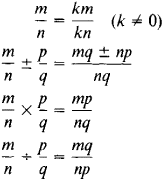# rational number

(redirected from Rationals)
Also found in: Dictionary, Thesaurus, Medical, Financial.
Related to Rationals: Radicals, Rational numbers, Real numbers

## rational number:

see numbernumber,
entity describing the magnitude or position of a mathematical object or extensions of these concepts. The Natural Numbers

Cardinal numbers describe the size of a collection of objects; two such collections have the same (cardinal) number of objects if their
..... Click the link for more information.
.
The Columbia Electronic Encyclopedia™ Copyright © 2013, Columbia University Press. Licensed from Columbia University Press. All rights reserved. www.cc.columbia.edu/cu/cup/
The following article is from The Great Soviet Encyclopedia (1979). It might be outdated or ideologically biased.

## Rational Number

a number that can be expressed in the form of a fraction m/n, where m and η are integers and n ≠ 0. Since an integer m can be expressed as m/1, all integers are rational numbers. The operations of addition, subtraction, multiplication, and division (by nonzero divisors) can always be performed in the domain of rational numbers; thus, the rational numbers form a field. The basic rules of the operations over the rational numbers are given by the formulasRational numbers can also be represented in the form of finite decimals or infinite periodic decimals. Every irrational number can be included between two rational numbers, the difference between which can be made arbitrarily small.

The Great Soviet Encyclopedia, 3rd Edition (1970-1979). © 2010 The Gale Group, Inc. All rights reserved.

## rational number

[′rash·ən·əl ′nəm·bər]
(mathematics)
A number which is the quotient of two integers.
McGraw-Hill Dictionary of Scientific & Technical Terms, 6E, Copyright © 2003 by The McGraw-Hill Companies, Inc.

## rational number

any real number of the form a/b, where a and b are integers and b is not zero, as 7 or 7/3
Collins Discovery Encyclopedia, 1st edition © HarperCollins Publishers 2005
References in periodicals archive ?
In this section we study the neutrosophic duplets of the neutrosophic rings <Q [union] I> = {a+bI|a, b [member of] Q, [I.sup.2] = I}; where Q the field of rationals and <R [union] I> = {a + bI|a, b, [member of] R, [I.sup.2] = I}; where R is the field of reals.
Because 5/3 CDF filter coefficients are dyadic rational values and so the integer to integer transform is obtained by using this feature .
The symbols N, Q, and R denote the sets of positive integers, rational and real numbers, respectively.
Along the way we will introduce a lagrangian that exists as a purely polynomial expression and removes the need for complicated nonanalytic measures and rational inverse matrix functions.
In general, though, discrete non-positive measures on R do not attain their norm; for instance, let [[q.sub.n]] be an enumeration of the rationals and let [MATHEMATICAL EXPRESSION NOT REPRODUCIBLE IN ASCII]; since Q is everywhere dense in R, there is no continuous function f such that f([q.sub.n]) = [(-1).sup.n], and so [mu] cannot attain its norm as a functional on C(R).
Topics follow the order of whole numbers, fractions, integers, rationals, and reals.
(71.) Although all real numbers can be defined as limits of infinite sequences of rationals, almost all of these numbers are noncomputable in the sense that they cannot be computed via any possible algorithm.
Extend Isabelle's computational features in direction of verfied Computer Algebra: simplification extended by algorithms beyond rewriting (cancellation of multivariate rationals, factorisation, partial fraction decomposition, etc), equation solving , integration, etc.
(i) [right arrow] (ii) Define [A.sub.r] = [h.sup.-1]([L.sup.I.sub.r]) and [B.sub.r] = [g.sup.- 1]([bar.R.sup.I.sub.r]), for all r [member of] Q(Q is the set of all rationals).
Note that it is known that the boundary complex of a simplicial polytope has the WLP over the rationals.
Kindled at one Flame The World of Rationals; one Spirit pour'd From Spirits [sic] awful Fountain; pour'd Himself Thro' all their Souls, but not in equal Stream, Profuse, or frugal of th' inspiring God (4.521-25) The caveat implies the gift of talents (Matt.

Site: Follow: Share:
Open / Close